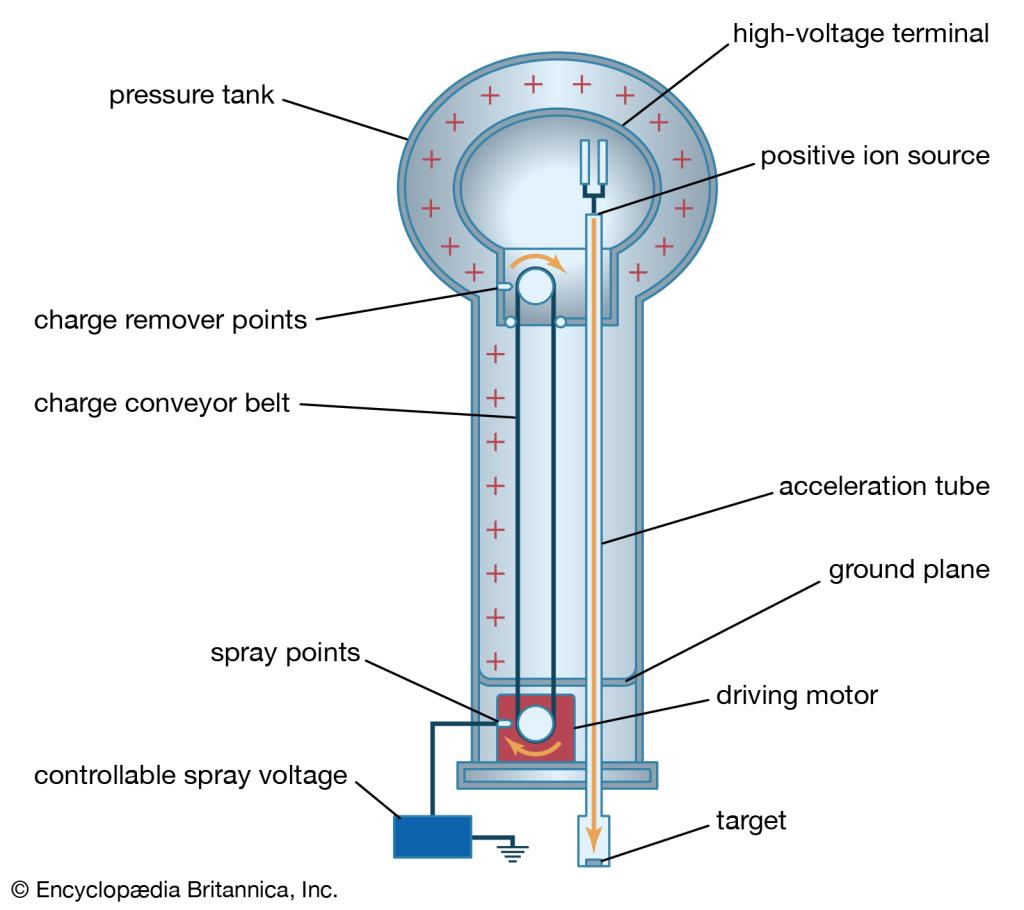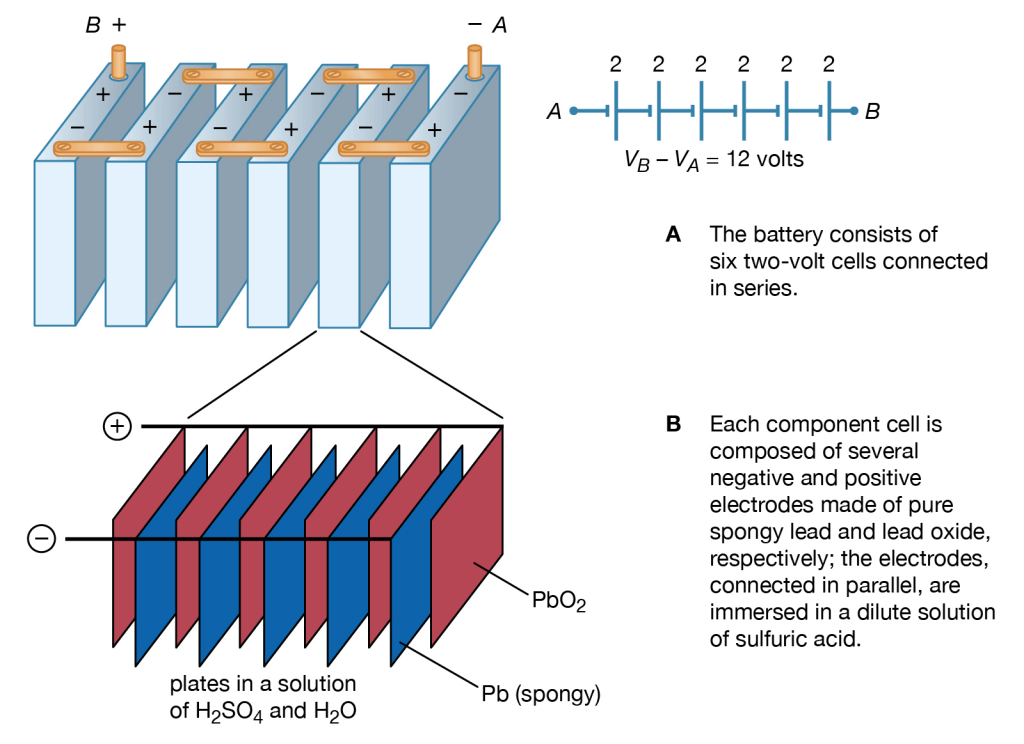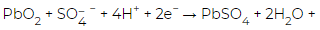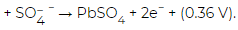Courses

# Electromotive Force Physics Notes | EduRev

## Physics : Electromotive Force Physics Notes | EduRev

The document Electromotive Force Physics Notes | EduRev is a part of the Physics Course Basic Physics for IIT JAM.
All you need of Physics at this link: Physics

A 12-volt automobile battery can deliver current to a circuit such as that of a car radio for a considerable length of time, during which the potential difference between the terminals of the battery remains close to 12 volts. The battery must have a means of continuously replenishing the excess positive and negative charges that are located on the respective terminals and that are responsible for the 12-volt potential difference between the terminals. The charges must be transported from one terminal to the other in a direction opposite to the electric force on the charges between the terminals. Any device that accomplishes this transport of charge constitutes a source of electromotive force. A car battery, for example, uses chemical reactions to generate electromotive force. The Van de Graaff generator shown in Figure is a mechanical device that produces an electromotive force. Invented by the American physicist Robert J. Van de Graaff in the 1930s, this type of particle accelerator has been widely used to study subatomic particles. Because it is conceptually simpler than a chemical source of electromotive force, the Van de Graaff generator will be discussed first.Figure 13: Van de Graaff accelerator.

An insulating conveyor belt carries positive charge from the base of the Van de Graaff machine to the inside of a large conducting dome. The charge is removed from the belt by the proximity of sharp metal electrodes called charge remover points. The charge then moves rapidly to the outside of the conducting dome. The positively charged dome creates an electric field, which points away from the dome and provides a repelling action on additional positive charges transported on the belt toward the dome. Thus, work is done to keep the conveyor belt turning. If a current is allowed to flow from the dome to ground and if an equal current is provided by the transport of charge on the insulating belt, equilibrium is established and the potential of the dome remains at a constant positive value. In this example, the current from the dome to ground consists of a stream of positive ions inside the accelerating tube, moving in the direction of the electric field. The motion of the charge on the belt is in a direction opposite to the force that the electric field of the dome exerts on the charge. This motion of charge in a direction opposite the electric field is a feature common to all sources of electromotive force.
In the case of a chemically generated electromotive force, chemical reactions release energy. If these reactions take place with chemicals in close proximity to each other (e.g., if they mix), the energy released heats the mixture. To produce a voltaic cell, these reactions must occur in separate locations. A copper wire and a zinc wire poked into a lemon make up a simple voltaic cell. The potential difference between the copper and the zinc wires can be measured easily and is found to be 1.1 volts; the copper wire acts as the positive terminal. Such a “lemon battery” is a rather poor voltaic cell capable of supplying only small amounts of electric power. Another kind of 1.1-volt battery constructed with essentially the same materials can provide much more electricity. In this case, a copper wire is placed in a solution of copper sulfate and a zinc wire in a solution of zinc sulfate; the two solutions are connected electrically by a potassium chloride salt bridge. (A salt bridge is a conductor with ions as charge carriers.) In both kinds of batteries, the energy comes from the difference in the degree of binding between the electrons in copper and those in zinc. Energy is gained when copper ions from the copper sulfate solution are deposited on the copper electrode as neutral copper ions, thus removing free electrons from the copper wire. At the same time, zinc atoms from the zinc wire go into solution as positively charged zinc ions, leaving the zinc wire with excess free electrons. The result is a positively charged copper wire and a negatively charged zinc wire. The two reactions are separated physically, with the salt bridge completing the internal circuit.
Figure 14 illustrates a 12-volt lead-acid battery, using standard symbols for depicting batteries in a circuit. The battery consists of six voltaic cells, each with an electromotive force of approximately two volts; the cells are connected in series, so that the six individual voltages add up to about 12 volts (Figure). As shown in Figure 14B, each two-volt cell consists of a number of positive and negative electrodes connected electrically in parallel. The parallel connection is made to provide a large surface area of electrodes, on which chemical reactions can take place. The higher rate at which the materials of the electrodes are able to undergo chemical transformations allows the battery to deliver a larger current.Figure 14: Voltaic cells and electrodes of a 12-volt lead-acid battery.

In the lead-acid battery, each voltaic cell consists of a negative electrode of pure, spongy lead(Pb) and a positive electrode of lead oxide (PbO2). Both the lead and lead oxide are in a solution of sulfuric acid (H2SO4) and water (H2O). At the positive electrode, the chemical reaction is(1.68 V). At the negative terminal, the reaction is Pb. The cell potential is 1.68 + 0.36 = 2.04 volts. The 1.68 and 0.36 volts in the above equations are, respectively, the reduction and oxidation potentials; they are related to the binding of the electrons in the chemicals. When the battery is recharged, either by a car generator or by an external power source, the two chemical reactions are reversed.

Offer running on EduRev: Apply code STAYHOME200 to get INR 200 off on our premium plan EduRev Infinity!

,

,

,

,

,

,

,

,

,

,

,

,

,

,

,

,

,

,

,

,

,

;